# PERFORMANCE OF COLLINEAR THERMOELECTRIC GENERATOR CONSIDERING THE HEAT DISSIPATION IN THE SIDE SURFACE1)

Wang Xueqiang, Ju Chengjian, Dui Guansuo2)School of Civil Engineering, Beijing Jiaotong University, Beijing 100044, China

Abstract

Thermoelectric material is an environment-friendly function material, which can~convert energy between heat and electricity. And it holds extensive~application~potentiality in power generation and refrigeration. The traditional thermoelectric generator is a $\pi$-type structure, which requires the length of the thermoelectric legs to be equal. In some cases, the structure is not conducive to the optimal design of the thermoelectric generator. Intense thermal stress and even stress~concentration will be induced in the thermoelectric generator due to high-temperature working condition, leading to shortening its working life. In addition, since the operating temperature of the thermoelectric generator is higher than ambient temperature, part of the heat will inevitably be dissipated to the environment, which will affect the thermoelectric performance and mechanical performance of the thermoelectric generator. Therefore, the heat dissipation cannot be neglected when analyzing this kind of problem. For these phenomena, in this work, a novel collinear-type thermoelectric generator model is proposed considering the heat dissipation in the side surface. And the legs of the proposed thermoelectric model can be optimized independently. Then, based on the finite element method, performance of collinear thermoelectric generator considering the heat dissipation in the side surface is simulated. And the thermoelectric performance and mechanical performance under the Dirichlet boundary condition is analyzed. Simultaneously, the temperature field, electric potential field and stress field in the thermoelectric generator are obtained. The influence of various convective heat transfer coefficient on thermoelectric performance and mechanical performance of the thermoelectric generator is investigated. The results demonstrate that thermal convection can decrease the energy conversion efficiency of the thermoelectric generator. When the convective heat transfer coefficient reaches 100 W/(m$^{2}\cdot$\textcelsius), the efficiency is 0.047 9 which is 28% lower than the conversion efficiency of 0.066 7 in adiabatic state. Though heat loss from the side surface is increased due to heat convection, thermal stress is reduced. In practical application, proper design and improvement of the thermal insulation system should be carried out to improve the efficiency of energy conversion.

Keywords： thermoelectric generator ; thermal convection ; finite element method ; thermoelectric performance ; mechanical performance

0

Wang Xueqiang, Ju Chengjian, Dui Guansuo. PERFORMANCE OF COLLINEAR THERMOELECTRIC GENERATOR CONSIDERING THE HEAT DISSIPATION IN THE SIDE SURFACE1)[J]. Chinese Journal of Theoretical and Applied Mechanics, 2019, 51(1): 192-197 https://doi.org/10.6052/0459-1879-18-255

## 引言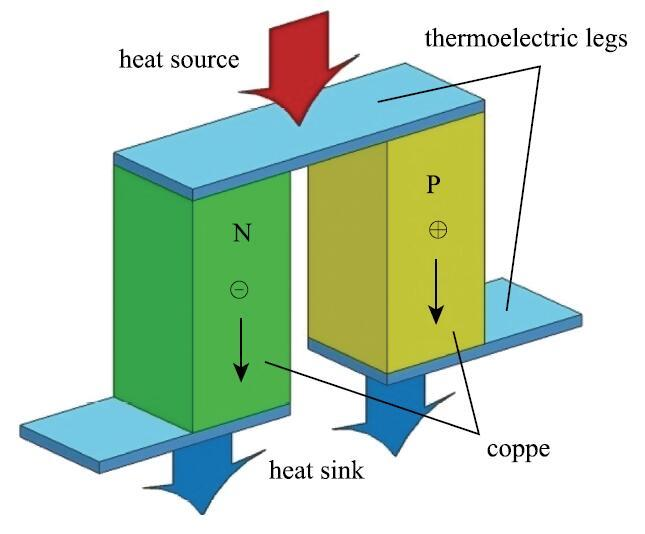Fig. 1   The traditional $\pi$-type TEG

## 1 模型与参数

### 1.1 物理模型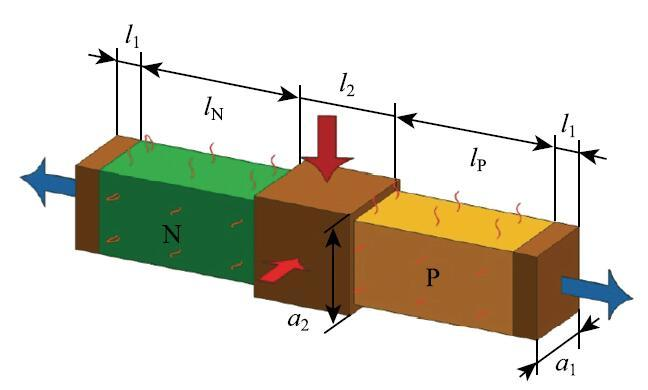Fig.2   The collinear-type TEG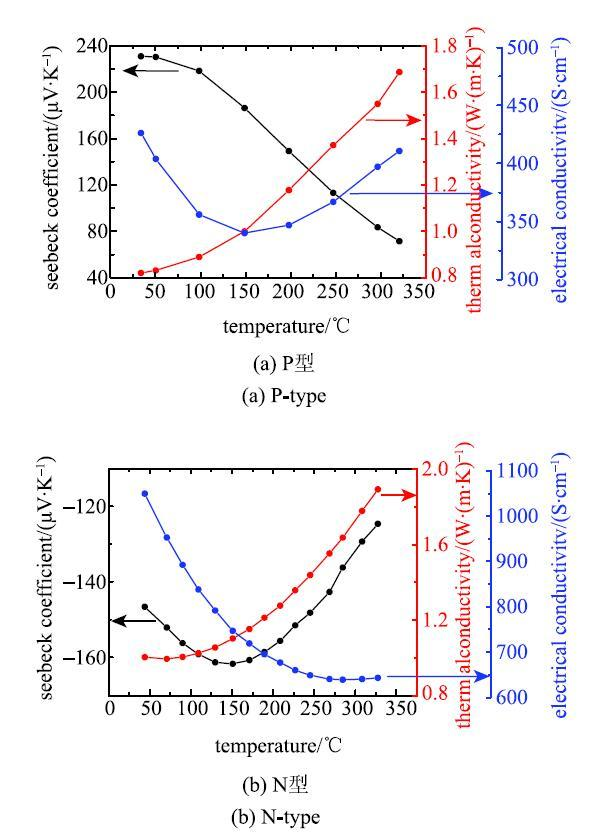Fig.3   Properties of the semiconductor materials with temperature-dependence

Table 1   Properties of the materials

MaterialsYoung’s
modulus/GPa
Poisson’s
ratio
Coefficient of thermal expansion/K-1
Bi2Te3450.221.6x10-5
copper1200.301.7x10-5

### 1.2 基本假设

(1) 忽略接触电阻和接触热阻。

(2) 两低温端面固定,其他边界为自由状态。

(3) 采用狄利克雷边界条件。

### 1.3 热力学模型

$$\begin{eqnarray} \label{eq1} \hspace{-8mm}&\hspace{-3mm}&\nabla \left( {k\nabla T} \right) \!+\! h\left( {T \!-\! T_0 } \right) \!+\! \rho J^2 \!-\! TJ \cdot \left[ {\left( {\nabla \alpha } \right)_T \!+\! \left( {\frac{\partial \alpha }{\partial T}} \right)\nabla T} \right] \!=\! 0\\ \label{eq2} \hspace{-8mm}&\hspace{-3mm}&\nabla \cdot \sigma \left[ {\nabla E + \alpha \nabla T} \right] = 0 \end{eqnarray}$$

\begin{equation} \label{eq3} E = \alpha \cdot \nabla T-\rho J \end{equation}

\begin{equation} \label{eq4} P = I^{2}R_{L} \end{equation} \begin{equation} \label{eq5} \eta = \frac{P}{Q_{H} } \end{equation}

## 2 结果与分析

===图8 显示了沿外侧上边缘线等效应力~(EQV) 和温度梯度的分布情况. 应力沿着中间横截面几乎对称分布,在靠近两边缘处,由于是完全固定约束的边界,物体的自由膨胀受到限制,因此应力较大. 在铜导流片与半导体腿结合面处,应力值较大,且出现峰值. 距离结合面和固定面越远,物体受到限制越小,应力逐渐减小. 在靠近中心处应力较小,这是因为中心处的温度梯度较小.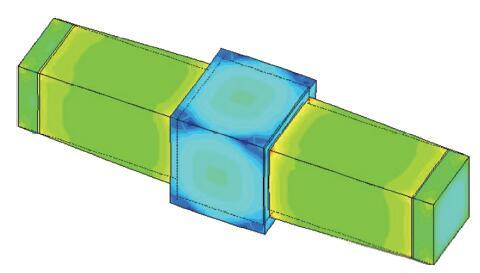Fig.7   Distributions diagram of stress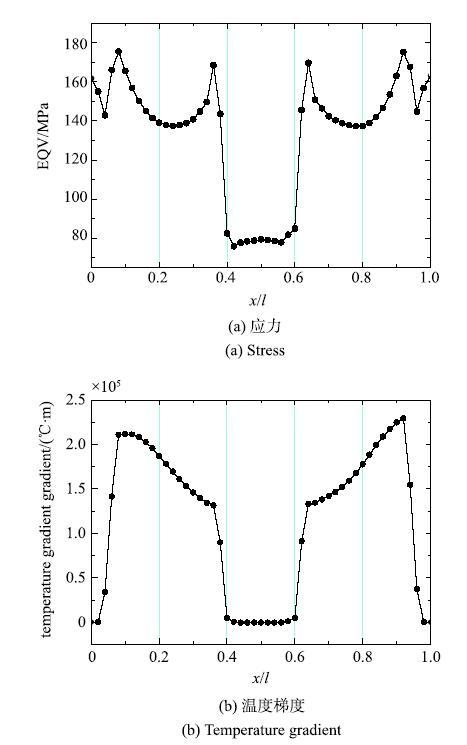Fig.8   Stress and temperature gradient curves at edge line

===图9 给出了组件的最大、最小应力随着对流换热系数的变化. 铜导流片和半导体腿的最大应力值均随着对流换热系数的增大而线性减小, 说明了对流换热降低了热电发电机的应力水平.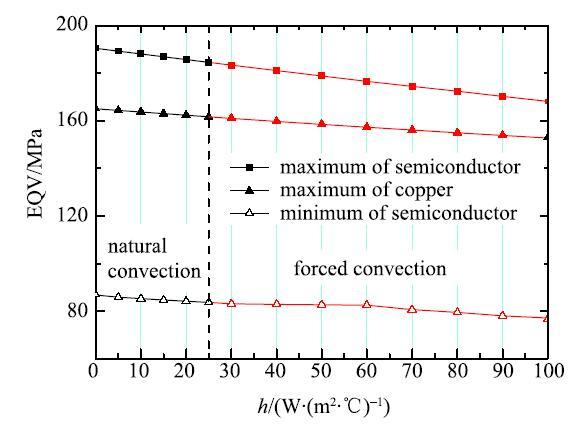Fig.9   Ultimate stress variation

## 3 结论

TEG的性能还会受到操作工况的影响,接下来的工作将对器件的瞬态特性进行研究.

The authors have declared that no competing interests exist.

## 参考文献 原文顺序 文献年度倒序 文中引用次数倒序 被引期刊影响因子

  Al-Nimr MA, Tashtoush BM, Khasawneh MA, et al.A hybrid concentrated solar thermal collector/thermo-electric generation system. Energy, 2017, 134: 1001-1012  Chen G.Theoretical efficiency of solar thermoelectric energy generators. Journal of Applied Physics, 2011, 109(10): 773-778  贾磊, 陈则韶, 胡芃等. 导体温差发电器件的热力学分析. 中国科学技术大学学报, 2004, 34(6): 684-687 (Jia Lei, Chen Zeshao, Hu Peng, et al.Thermodynamic analysis of semiconductor thermoelectric generator. Journal of University of Science and Technology of China, 2004, 34(6): 684-687 (in Chinese))  Hadjistassou C, Kyriakides E, Georgiou J.Designing high efficiency segmented thermoelectric generators. Energy Conversion and Management, 2013, 66: 165-172  Kim S.Analysis and modeling of effective temperature differences and electrical parameters of thermoelectric generators. Applied Energy, 2013, 102(2): 1458-1463  贾阳, 任德鹏. 温差发电器中热电材料物性的影响分析. 电源技术, 2008, 32(4): 252-256 (Jia Yang, Ren Depeng.Effect analysis for physics characteristic of thermo-electric materials in thermo-electric generator. Journal of Power Sources, 2008, 32(4): 252-256 (in Chinese))  Ju CJ, Dui GS, Zheng H, et al.Revisiting the temperature dependence in material performance and performance of thermoelectric materials. Energy, 2017, 124: 249-257  Wang BL.A finite element computational scheme for transient and nonlinear coupling thermoelectric fields and the associated thermal stresses in thermoelectric materials. Applied Thermal Engineering, 2017, 110: 136-143  杜群贵, 邹杰慧, 陈水金等. 半导体热电转换单元发电性能的变物性计算模型. 华南理工大学学报, 2013, 41(4): 47-53 (Du Qungui, Zou Jiehui, Chen Jinshui, et al.Calculation model of semiconductor thermoelectric generator unit based on variable material properties. Joumal of South China University of Technology, 2013, 41(4): 47-53 (in Chinese))  Liu ZC, Zhu SP, Ge Y, et al.Geometry optimization of two-stage thermoelectric generators using simplified conjugate-gradient method. Applied Energy, 2017, 190: 540-552  Ali H, Sahin AZ, Yilbas BS.Thermodynamic analysis of a thermoelectric power generator in relation to geometric configuration device pins. Energy Conversion and Management, 2014, 78: 634-640  Al-Merbati AS, Yilbas BS, Sahin AZ.Thermodynamics and thermal stress analysis of thermoelectric power generator: Influence of pin geometry on device performance. Applied Thermal Engineering, 2013, 50: 683-692  Sahin AZ, Yilbas BS.The thermoelement as thermoelectric power generator: Effect of leg geometry on the efficiency and power generation. Energy Conversion Management, 2013, 65: 26-32  Kim H S, Itoh T, Iida T, et al.Design of linear shaped thermoelectric generator and self-integration using shape memory alloy. Materials Science and Engineering: B, 2014, 183(1): 61-68  Jia XD, Gao YW.Optimal design of a novel thermoelectric generator with linear-shaped structure under different operating temperature conditions. Applied Thermal Engineering, 2015, 78: 533-542  Jia XD, Wang YJ, Gao YW.Numerical simulation of thermoelectric performance of linear-shaped thermoelectric generators under transient heat supply. Energy, 2017, 130: 276-285  Yilbas BS, Akhtar SS, Sahin AZ.Thermal and stress analyses in thermoelectric generator with tapered and rectangular pin configurations. Energy, 2016, 114: 52-63  王长宏, 李娜, 林涛等. 半导体~P-N 型温差发电器件热电性能研究. 功能材料, 2016, 47(12): 12147-12151 (Wang Changhong, Li Na, Lin Tao, et al.Research on thermoelectric properties of semiconductor P-N type thermoelectric power generation device. Functional Materials, 2016, 47(12): 12147-12151 (in Chinese))  张克实, 黄世鸿, 刘贵龙等. 纯铜后继屈服面的测试与晶体塑性模型模拟. 力学学报, 2017, 49(4): 870-879 (Zhang Keshi, Huang Shihong, Liu Guilong, et al.Measuring subsequent yield surface of pure copper by crystal plasticity simulation. Chinese Journal of Theoretical and Applied Mechanics, 2017, 49(4): 870-879 (in Chinese))  Drink E, Martin J, Markus B, et al.Multiphysics simulation of thermoelectric systems for comparison with experimental device performance. Journal of Electronic Materials, 2009, 38(7): 1456-146  龙凯, 王选, 韩丹. 基于多相材料的稳态热传导结构轻量化设计. 力学学报, 2017, 49(2): 359-366 (Long Kai, Wang Xuan, Han Dan.Structural light design for steady heat conduction using multi-material. Chinese Journal of Theoretical and Applied Mechanics, 2017, 49(2): 359-366 (in Chinese))  章鹏, 杜成斌, 江守燕. 比例边界有限元法求解裂纹面接触问题. 力学学报, 2017, 49(6): 1335-1347 (Zhang Peng, Du Chengbin, Jiang Shouyan.Crack face contact problem analysis using the scaled boundary finite element method. Chinese Journal of Theoretical and Applied Mechanics, 2017, 49(6): 1335-1347 (in Chinese)/

 〈〉